# Multiplying Square Roots: 3 Easy Methods [with Examples]

Jordan Nisbet

All Posts

Your students know how to multiply exponents, but now it’s time to teach them about multiplying square roots and the wonderful world of pre-algebra. But, you fear they might be thinking: “In math class, we learned more about algebra, such as X + 10 = Y, but why should I care?”

You want them to realize what French mathematician, Jean de Rond d’Alembert, famously said: “algebra is generous; she often gives more than is asked of her.”

Just because you don’t see Xs and Ys doesn’t mean you aren’t using algebra every day. Learning how to multiply square roots is one cobblestone on the scenic road to understanding algebra’s real-life relevance.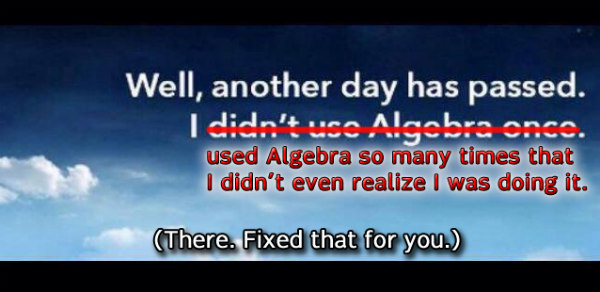Educators like yourself know it’s not always easy to make these abstract and complex concepts engaging and fun.

This blog post — divided into three parts — aims to change that!

• Understanding square roots
• How to multiply square roots
• Engaging ways to enforce students’ square root knowledge

## Part One: What is a “square root”?

The square root of a number refers to the factor you can multiply by itself to get that number. Put another way, finding the square root is the opposite process of squaring a number.

It’s only possible to take the square root of non-negative numbers — even ones that don’t yield whole numbers. This is because any number times itself is positive, or zero — you can never get a negative product when you square a negative number. Like you saw above, square roots undo squaring, so it’s impossible for negative numbers to have square roots.

That said, perfect square numbers are the most effective ones to use when teaching students about multiplying square roots.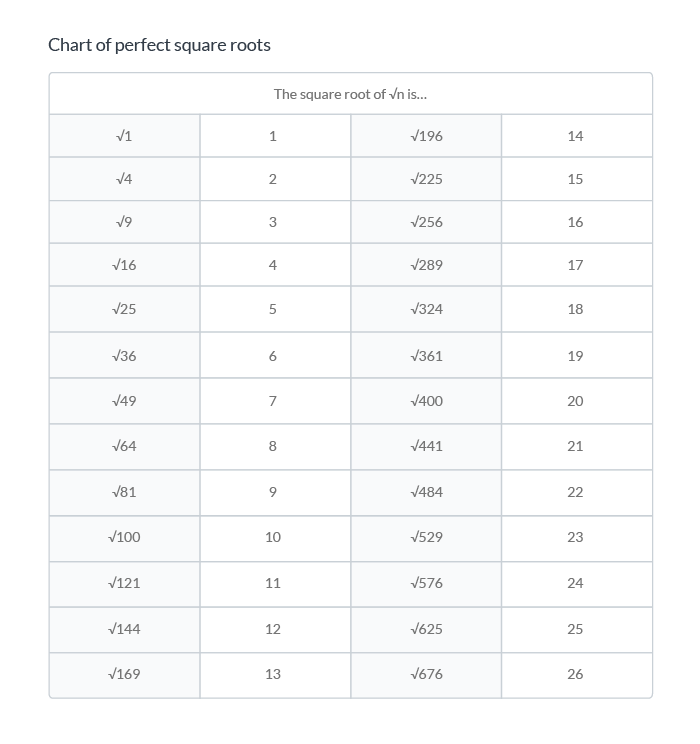In the visual below we see the square root of 16 is 4 because 4 squared, or 42, is 16.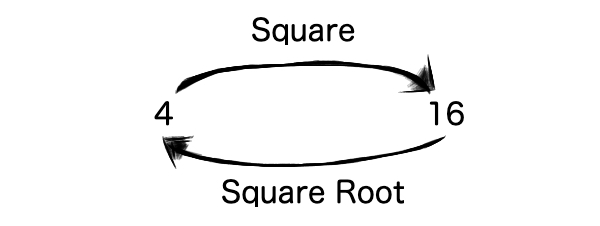In math class, this kind of equation would look like:Students seeing this for the first time will likely have some questions: What’s that check mark-looking symbol? Why’s there a tiny number over it?

Brett Berry, the founder of Math Hacks, created a clear and concise image with all the root terminology in her article on understanding logarithms and roots.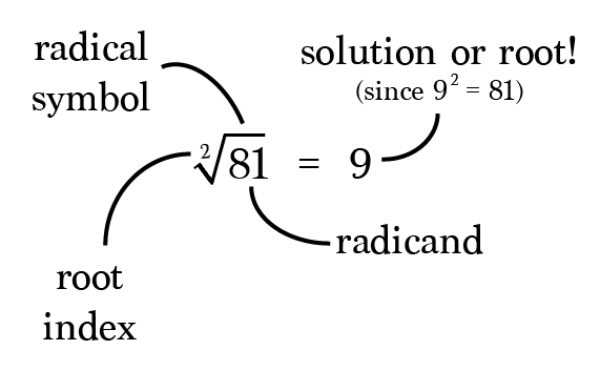Many questions about finding square roots don’t include the root index. However, root indexes are necessary when calculating higher indexed roots like cube, fourth, or fifth roots.

### When will I ever need to multiply square roots?

No matter how well you teach these algebraic concepts, students will always ask that question. ☝️

And you should be prepared to provide them with legitimate answers. For example, multiplying square roots can be important for:

• Knowing the square footage of their future homes
• Architects
• Artists
• Carpenters
• Construction workers
• Designers
• Engineers

There are more, too! While some will have to calculate equations every day, others will use the concepts to make estimates. One thing’s for sure — people in those professions learned math in school as kids and they’re still using it today!

## Part Two: 3 Simple methods for multiplying square roots

### Multiplying square roots without coefficients

1. Multiply each radicand the same way you would without the radical, or square root symbol.2.Simplify the radicand by factoring out all perfect squares. If there are no perfect squares in the radicand, it’s already simplified. In this case, you can simplify √98 to √2 and √49 — a perfect square.3. Take out the square root of the perfect square. In this example, simplify √49 to 7 and place it in front of the remaining expression, √2.### Multiplying square roots with coefficients

1. Multiply coefficients in front of radical signs, if any.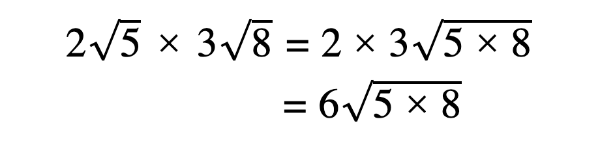2.Multiply each radicand the same way you would without the radical, or square root symbol.3. Simplify the radicand by factoring out all perfect squares. In this example, you can simplify √40 to √4 and √10.4. Take out the square root of the perfect square and multiply it by the coefficient. In the example, simplify √4 to 2 and multiply it by 6.### Multiplying square roots with variables

In addition to numbers, radicals can contain other things like variables and exponents. Simplifying radicals with variables follows the same rules as one that only has numbers.

1. Multiply the radicands. If there are coefficients multiply them together as well.Note: In order to multiply radicals containing variables, the:

• Root index must be the same
• Value of x — along with any other variables — must be greater than or equal to zero

2. Find the prime factorizations to identify the perfect square factors. To do this, you can use a factor tree — like in the image below.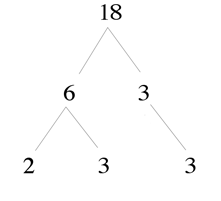For example, 2 and 9 equal 18, and 9 simplifies further to 3 and 3. So, the prime factorizations and perfect square factors for 18 would be 2, 3, and 3. The prime factorization of 30 is 2, 3, and 5. You can also break down and rearrange the variable exponents — you can rewrite x3 as x2 and x.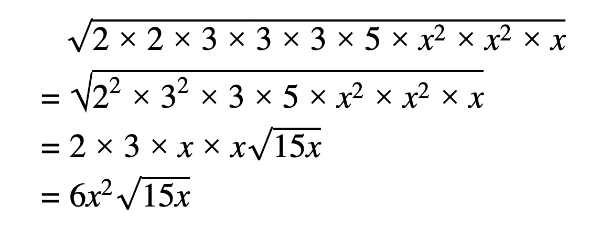## Part Three: Activities to enforce students’ square root knowledge

After teaching your students about square roots — and how to multiply them — split them into groups to make some radical number towers! Starting with √1, have them make a tower going all the way up to the square root of your choice.

On or beside each part of the tower, you can have students write both the square and square root. This activity is an effective visual representation of what each one looks like and should help them remember the most common square roots.

Lisa Tarman, an educator from Pennsylvania, has created hundreds of teaching resources. Her “Simplifying Radicals Maze” is a refreshing and engaging take on traditional worksheets.

Instruct your students to start in the top left corner. They’ll have to simplify radicals in order to make their way to the end of the maze. Access Tarman’s free fun worksheet and answer key here.

If you want a multiplying radicals maze, check out this one from Teachers Pay Teachers!

### Play ProdigyProdigy is a no-cost, adaptive math game used by one and a half million teachers and more than 50 million students around the world! It offers content from every major math topic and covers 1st to 8th grade, including how to:

• Evaluate perfect roots
• Rewrite exponents as roots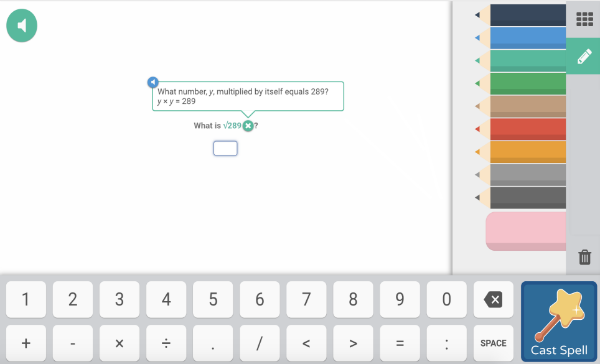Using Prodigy in your classroom will help students build math fluency and confidence in future high school and college-level math courses. Your class will explore a world filled with exciting quests that deliver personalized, curriculum-aligned content and real-time student data.

With these methods and activities in mind, you can see how multiplying square roots doesn’t have to remain irrelevant or intimidating for you or your students.

When used effectively, activities like the ones above can help solidify students’ understanding and create levels of engagement teachers seldom witness in math class.

Are you an educator? Customize math questions to supplement class material and differentiate instruction, addressing each student’s trouble spots.

Prodigy also features powerful and immediate reporting tools for both teachers and parents. From Progress Reports to Usage Reports and more, use your student or child’s data to identify where they’re excelling or struggling so you can adjust the content in game for them.

Click here or the banner below to get start in less than five minutes!•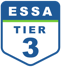•••